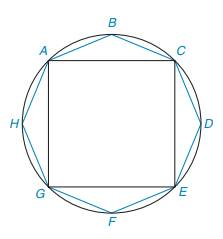Chapter 8.3, Problem 36EElementary Geometry For College St...

7th Edition
Alexander + 2 others
ISBN: 9781337614085

Solutions

Chapter
SectionElementary Geometry For College St...

7th Edition
Alexander + 2 others
ISBN: 9781337614085
Textbook Problem

Regular octagon ABCDEFGH is inscribed in a circle whose radius is 7 2 2 cm. Considering that the area of the octagon is less than the area of the circle and greater than the area of the square ACEG, find the two integers between which the area of the octagon must lie.(Note: For the circle, use A = π r 2 with π ≈ 22 7 .)To determine

To find:

The two integers between which the area of the octagon must lie.

Explanation

1) The diameter of the circle is twice the radius.

2) The area of the circle is given by, A=πr2 where r is the radius of the circle.

3) The area of the square is given by, A=d22 where d is diagonal of the square.

Calculation:

It is given that the radius of the circle is 722cm.

Use the formula to find the area of the circle, A=πr2

Substitute r=722 in A=πr2

Acircle=π(722)2=49π2=492×227=77cm2

The diameter of the circle is given by 72cm

Still sussing out bartleby?

Check out a sample textbook solution.

See a sample solution

The Solution to Your Study Problems

Bartleby provides explanations to thousands of textbook problems written by our experts, many with advanced degrees!

Get Started

Find more solutions based on key concepts

Elementary Technical Mathematics

In Exercises 2730, determine all values of x for which each function is discontinuous. 28. f(x) = 3x+44x22x2

Applied Calculus for the Managerial, Life, and Social Sciences: A Brief Approach

In Exercises 516, evaluate the given quantity. log5125

Finite Mathematics and Applied Calculus (MindTap Course List)

The horizontal asymptote(s) for f(x)=|x|x+1 is (are): a) y =0 b) y = 1 c) y = 1 d) y = 1 and y = 1

Study Guide for Stewart's Single Variable Calculus: Early Transcendentals, 8th